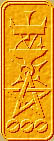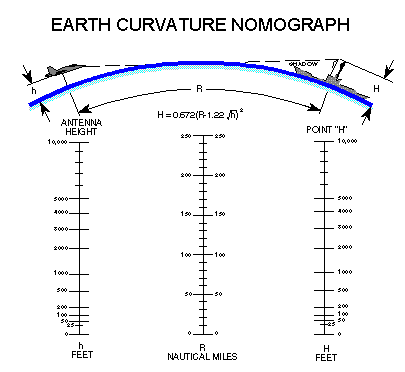Nomography Main > Parallel Scale Detailsρ=Σ+Ψ
``` <!-- RegisterNomoPage("3varaddB.html") PrintNomoHeader("Reference", "Antique Instruments"); PrintNomoPageNum() //--> ```

# Parallel Scale Nomograms In Depth

Naturally one will desire to make a nomogram that does something more awe inspiring than simple addition or multiplication.

When you do, you will probably want to play with the functional moduli of the various scales. This will allow one to do things like magnify one scale for better accuracy and related tricks.

If you peek at the cheat sheet for parallel scale nomograms you will discover that there are four functional moduli: δ1, δ3, μ1, and μ3. The δ's scale the x axis spacing and the μ's scale the y axis. You can choose any values for these you want, as long as the following is true: δ1331

As I will show you later, the easy way is to have the entire nomogram in a spreadsheet, so you can play with the functional moduli until the nomogram looks the way you want.

This is the "Constructional Determinant" of a parallel line nomogram from the cheat sheet:

 -δ1 μ1 . f(u) 1 0 -{{μ1 . μ3} / {μ1+μ3}} . f(v) 1 δ3 μ3 . f(w) 1

It will be explained in the section on Determinants. For now, here it is translated into something you can actually use:

 X coordequation Y coordequation U scale -δ1 μ1 * f(u) V scale 0 -{{μ1 * μ3} / {μ1+μ3}} . f(v) W scale δ3 μ3 * f(w)

Professor Allcock uses U, V and W instead of A, B, and C. Deal with it.

Remember, parallel scale charts can be used for any equation in the form f(u) + f(v) + f(w) = 0 where the three "f( )" are functions, and u, v, w are variables.

Take the equation:

H = 0.672 * ( R - ( 1.22 * sqrt[h] ) )^2

where
sqrt[x] means take the square root of x
x^2 means x squared

Given the height in feet h of your radar dish and the distance in nautical miles R it will calculate the maximum height in feet H an enemy object can be and still be just out of sight over the curvature of the earth.

Using simple algebra,

H = 0.672 * ( R - ( 1.22 * sqrt[h] ) )^2

can be re-written into the form f(u) + f(v) + f(w) = 0 as

( 1.22 * sqrt[h] ) - R + sqrt[H / 0.672] = 0 (I warned you that the fancier nomograms required algebra!)

This means:

f(u) = ( 1.22 * sqrt[h] )

f(v) = -R

f(w) = sqrt[H / 0.672]

Substituting them into the matrix gives us:

 X coordequation Y coordequation U scale -δ1 μ1 * ( 1.22 * sqrt[h] ) V scale 0 -( ( μ1 * μ3 ) / ( μ1+μ3 ) ) * -R W scale δ3 μ3 * sqrt[H / 0.672]

It is easiest if the distance between the scales are the same. This means δ1 = δ3 Glancing at the modulus constraints δ1331 it will tell you that if δ1 = δ3 then μ1 = μ3. So if we set δ1 = δ3 the formula simplifies to:

 X coordequation Y coordequation U scale -δ μ * ( 1.22 * sqrt[h] ) V scale 0 ( ( μ^2 ) / ( μ*2 ) ) * R W scale δ μ * sqrt[H / 0.672]
(a negative times a negative is a positive, this simplifies the V scale y coord equation)

Say we make δ = 40 mm and μ = 5 mm, the nomogram will be about 8 cm wide by 6.25 cm tall ( since the width is 2 * δ and I've cheated and found by experiment that if the u scale is from 0 to 10,000 it will be 125 units tall). The formula further simplifies to:

 X coordequation Y coordequation U scale -40 5 * 1.22 * sqrt[h] V scale 0 2.5 * R W scale 40 5 * sqrt[H / 0.672]
or
 X coordequation Y coordequation U scale -40 6.1 * sqrt[h] V scale 0 2.5 * R W scale 40 6.1 * sqrt[H]